Courses

# Past Year Questions: Static, Dynamic Loading, And Failure Theories Notes | EduRev

## Mechanical Engineering : Past Year Questions: Static, Dynamic Loading, And Failure Theories Notes | EduRev

The document Past Year Questions: Static, Dynamic Loading, And Failure Theories Notes | EduRev is a part of the Mechanical Engineering Course Machine Design.
All you need of Mechanical Engineering at this link: Mechanical Engineering

Question 1:A machine element is subjected to the following bi-axial state of stress; σx = 80 MPa; σy = 20 MPa; τxy = 40 MPa. If the shear strength of the material is 100 MPa, the factor of safety as per Tresca's maximum shear stress theory is

[2015, Set-1]

Question 2:Consider the two states of stress as shown in configurations I and II in the figure below.
From the standpoint of distortion energy (vonMises) criterion, which one of the following statements is true?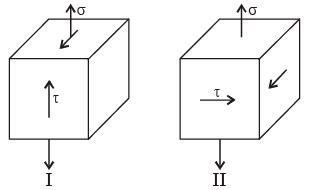[2014, Set-2]

Question 3:Which one of the following is not correct?

[2014, Set-3]

Question 4:Which theory of failure will you use for aluminium components under steady loading is



Question 5:A small element at the critical section of a component in biaxial state of stress with the two principal stresses being 360 MPa and 140 MPa. The maximum working stress according to distortion energy theory is



Question 6:In the design of shafts made of ductile materials subjected to twisting moment and bending moment, the recommended theory of failure is



Question 7:Consider the schematic of a riveted lap Joint subjected to tensile load F, as shown below.
Let d be the diameter of the rivets, and Sf be the maximum permissible tensile stress in the plates. What should be the minimum value for the thickness of the plates to guard against tensile failure of the plates? Assume the plates to be identical.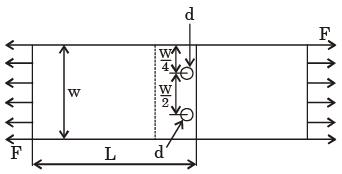[2017, Set-1]

Question 8:For the given fluctuating fatigue load, the values of stress amplitude and stress ratio are respectively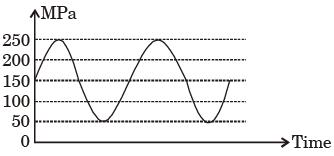[2015, Set-3]

Question 9:A bar is subjected to fluctuating tensile load from 20 kN to 100 kN. The material has yield strength of 240 MPa and endurance limit in reversed bending is 160 MPa. According to the Soderberg principle, the area of cross-section in mm2 of the bar for a factor of safety of 2 is



Question 10:A forged steel link with uniform diameter of 30 mm at the centre is subjected to an axial force that varies from 40 kN in compression to 160 kN in tension. The tensile (Su), yield (Sy) and corrected endurance (Se) strengths of the steel material are 600 MPa, 420 MPa and 240 MPa respectively. The factor of safety against fatigue endurance as per Soderberg's criterion is



Question 11:The piston rod of diameter 20 mm and length 700 mm in a hydraulic cylinder is subjected to a compressive force of 10 kN due to the internal pressure. The end conditions for the rod may be assumed as guided at the piston end and hinged at the other end. The Young's modulus is 200 GPa. The factor of safety for the piston rod is



Question 12:A thin spherical pressure vessel of 200 mm diameter and 1 mm thickness is subjected to an internal pressure varying from 4 to 8 MPa.Assume that the yield, ultimate, and endurance strength of material are 600,800 and 400 MPa respectively. The factor of safety as per Goodman's relation is



Question 13:A carpenter glues a pair of cylindrical wooden logs by bonding their end faces at an angle of θ = 30° as shown in the figure.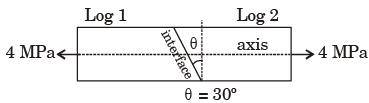The glue used at the interface fails if
Criterion 1 : the maximum normal stress exceeds 2.5 MPa
Criterion 2 : the maximum shear stress exceeds 1.5 MPa
Assume that the interface fails before the logs fail. When a uniform tensile stress of 4 MPa is applied, the interface

[2018, Set-1]

Question 14:If σ1 and σ3 are the algebraically largest and smallest principal stresses respectively, the value of the maximum shear stress is

[2018, Set-1]

Question 15:A pin jointed uniform rigid rod of weight W and length L is supported horizontally by an external force F as shown in the figure below.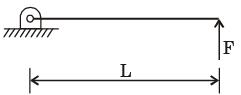The force F is suddenly removed. At the instant of force removal, the magnitude of vertical reaction developed at the support is



Question 16:A solid shaft can resist a bending moment of 3.0 kNm and a twisting moment of 4.0 kNm together, then the maximum torque that can be applied is



Question 17:Two shafts A and B are made of the same material. The diameter of shaft B is twice that of shaft A The ratio of power which can be transmitted by shaft A to that of shaft B is



Question 18:The outside diameter of a hollow shaft that is twice its inside diameter the ratio of its torque carrying capacity to that of a solid shaft of the same material and the same outside diameter is



Question 19:A large uniform plate containing a rivet hole subjected to uniform uniaxial tension of 95 MPa.
The maximum stress in the plate is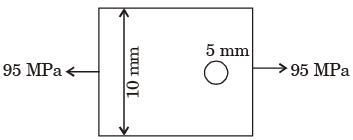Question 20:Torque to weight ratio for a circular shaft transmitting power is directly proportional to the



Offer running on EduRev: Apply code STAYHOME200 to get INR 200 off on our premium plan EduRev Infinity!

## Machine Design

57 videos|71 docs|102 tests

,

,

,

,

,

,

,

,

,

,

,

,

,

,

,

,

,

,

,

,

,

,

,

,

,

,

,

;